# Mutual Inductance

## AC Electric Circuits

• #### Question 1

When an electric current is passed through a coil of wire, what phenomenon occurs?

• #### Question 2

When a coil of wire “cuts through” magnetic lines of flux, what phenomenon occurs?

• #### Question 3

If one coil of wire is located very close to another coil of wire, and an electric current is passed through the first coil whose magnitude changes over time, what phenomenon will occur in the second coil of wire?

• #### Question 4

Write the equation describing the voltage induced in this coil, solving for (instantaneous) induced voltage (e) in terms of the instantaneous magnetic flux (φ) and the number of turns of wire in the coil: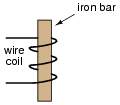• #### Question 5

There are two wire windings wrapped around a common iron bar in this illustration, such that whatever magnetic flux may be produced by one winding is fully shared by the other winding: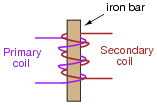Write two equations describing the induced voltage at each winding (ep = ... and es = ...), in each case expressing the induced voltage in terms of the instantaneous magnetic flux (φ) and the number of turns of wire in that winding (Np and Ns, respectively).

Then, combine these two equations, based on the fact that the magnetic flux is equal for each winding.

• #### Question 6

There are two wire windings wrapped around a common iron bar in this illustration, such that whatever magnetic flux may be produced by one winding is fully shared by the other winding:Write two equations describing the induced voltage at each winding (ep = ... and es = ...), in each case expressing the induced voltage in terms of the instantaneous current through that winding (ip and is, respectively) and the inductance of each winding (Lp and Ls, respectively).

We know that the induced voltages in the two windings are related to each other by this equation, if there is perfect “coupling” of magnetic flux between the two windings:

 ep Np = es Ns

Knowing this, write two more equations describing induced voltage, this time expressing the induced voltage in each winding in terms of the instantaneous current in the other winding. In other words,

 ep = ... is

 es = ... ip

• #### Question 7

Suppose two wire coils are wound around a common iron core, the “primary” coil with 100 turns of wire and the “secondary” coil with 300 turns of wire: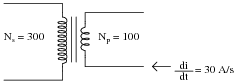If the inductance of the primary coil is 2 H, what is the inductance of the secondary coil, assuming that it “sees” the exact same magnetic circuit as the first coil (same permeability, same cross-sectional area, same length)?

If an electric current changing at a rate of 30 amps per second passes through the primary coil, how much voltage will be induced in each coil?

If only half the lines of magnetic flux from the primary coil “coupled” with the secondary coil, how much voltage would be induced in the secondary coil, given a primary current rate-of-change of 30 amps per second?

• #### Question 8

Mutual inductance is the term given to the phenomenon where a change in current through one inductor causes a voltage to be induced in another. When two inductors (L1 and L2) are magnetically “coupled,” the mutual inductance (M) relates their voltages and currents as such:

 e1 = M di2 dt Voltage induced in coil 1 by change of current in coil 2

 e2 = M di1 dt Voltage induced in coil 2 by change of current in coil 1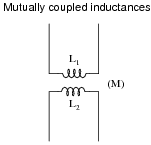When the magnetic coupling between the two inductors is perfect (k = 1), how does M relate to L1 and L2? In other words, write an equation defining M in terms of L1 and L2, given perfect coupling.

Hint:

 e1 = L2 N1 N2 di2 dt

 e2 = L1 N2 N1 di1 dt

 L1 L2 = ( N1 N2 ) 2

• #### Question 9

The coefficient of magnetic coupling between two coils affects the amount of mutual inductance between those two coils. This fact should be obvious, as coils not sharing any magnetic flux (k = 0) cannot have any mutual inductance between them.

Write an equation defining M in terms of L1 and L2, when k is something less than 1.

• #### Question 10

Mutual inductance can exist even in places where we would rather it not. Take for instance the situation of a “heavy” (high-current) AC electric load, where each conductor is routed through its own metal conduit. The oscillating magnetic field around each conductor induces currents in the metal conduits, causing them to resistively heat (Joule’s Law, P = I2 R):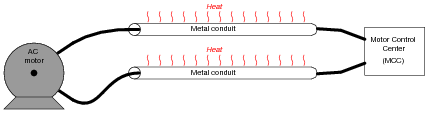It is standard industry practice to avoid running the conductors of a large AC load in separate metal conduits. Rather, the conductors should be run in the same conduit to avoid inductive heating: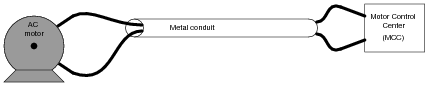Explain why this wiring technique eliminates inductive heating of the conduit.

Now, suppose two empty metal conduits stretch between the location of a large electric motor, and the motor control center (MCC) where the circuit breaker and on/off “contactor” equipment is located. Each conduit is too small to hold both motor conductors, but we know we’re not supposed to run each conductor in its own conduit, lest the conduits heat up from induction. What do we do, then?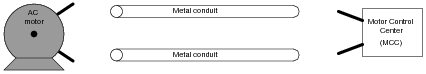• #### Question 11

Suppose a technician needs 167 mH of inductance in a circuit, but only has 500 mH and 250 mH inductors on hand. He decides he should be able to achieve approximately 167 mH of inductance easily enough by connecting these two inductors in parallel with one another on a printed circuit board: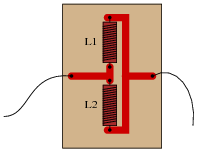However, upon testing this parallel inductor arrangement, the technician finds the total inductance to be significantly less than the 167 mH predicted. Puzzled, he asks a fellow technician for help. The other technician inspects the board, and immediately suggests that the two inductors be re-located with their axes perpendicular to one another. The first technician doesn’t understand why the physical location of the inductors should matter. After all, it never mattered how he located resistors and capacitors with respect to one another, so long as their connecting wires (or board traces) went to the right places. Can you explain to him why inductors might be sensitive to physical orientation?